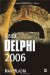Accessing Array Elements

Pointers can be used to access array elements. To read the address of the first element in the array (to point to the first element), you can write this:

var   Numbers: array[1..20] of Integer;   PI: PInteger; begin   PI := @Numbers; end.

To point to another element in the array, simply increment the pointer using the Inc procedure. However, you also need to typecast the pointer to an integer before passing it to the Inc procedure:

Inc(Integer(PI)); { move to next element }

The Inc procedure doesn't increment the pointer by one but by SizeOf(ElementType). In this case, the Inc procedure increments the pointer by 4 (SizeOf(Integer)), thus moving 4 bytes farther into memory, to the correct address at which the next array element is stored. Listing 9-4 shows how to access array elements through a pointer.

Listing 9-4: Accessing an array through a pointerprogram Project1; {\$APPTYPE CONSOLE} uses   SysUtils; var   Numbers: array[1..20] of Integer;   I: Integer;   PI: PInteger; begin   { standard access }   for I := Low(Numbers) to High(Numbers) do   begin     Numbers[I] := I;     WriteLn(Numbers[I]);   end;   { pointer access }   PI := @Numbers;          { point to first element in Numbers }   for I := Low(Numbers) to High(Numbers) do   begin     PI^ := I;     WriteLn(PI^);     Inc(Integer(PI));         { move to next element }   end;   ReadLn; end.You can also use pointers to access characters in a string. The following code shows the standard way of accessing characters in a string:

var   s: string;   i: Integer; begin   s := 'Borland Delphi';   for i := 1 to Length(s) do     Write(s[i]); end.

When you access characters in a string through a pointer, you don't have to call the Length function to determine the length of the string nor do you have to use the counter variable to index the characters in the string.Inside Delphi 2006 (Wordware Delphi Developers Library)
ISBN: 1598220039
EAN: 2147483647
Year: 2004
Pages: 212